Question

# Margin of Safety a. If Canace Company, with a break-even point at \$547,800 of sales, has...

Margin of Safety

a. If Canace Company, with a break-even point at \$547,800 of sales, has actual sales of \$830,000, what is the margin of safety expressed (1) in dollars and (2) as a percentage of sales? Round the percentage to the nearest whole number.

1. \$

2.   %

b. If the margin of safety for Canace Company was 40%, fixed costs were \$1,413,600, and variable costs were 60% of sales, what was the amount of actual sales (dollars)?
(Hint: Determine the break-even in sales dollars first.)
\$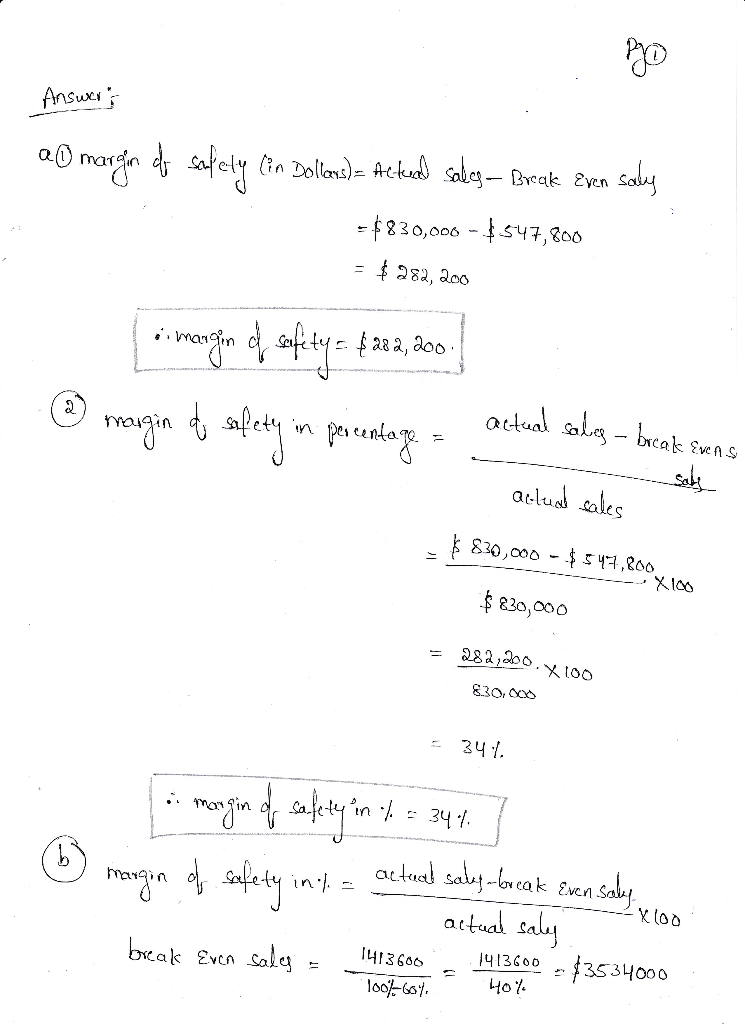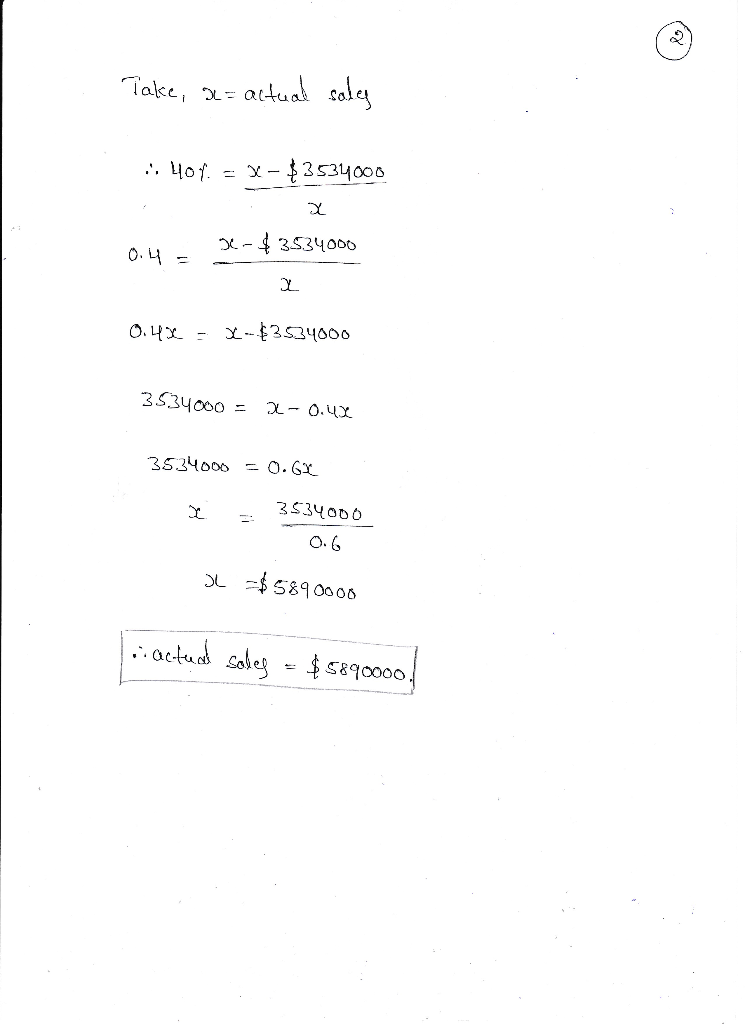#### Earn Coins

Coins can be redeemed for fabulous gifts.

Similar Homework Help Questions
• ### Margin of Safety a. If Canace Company, with a break-even point at \$396,000 of sales, has...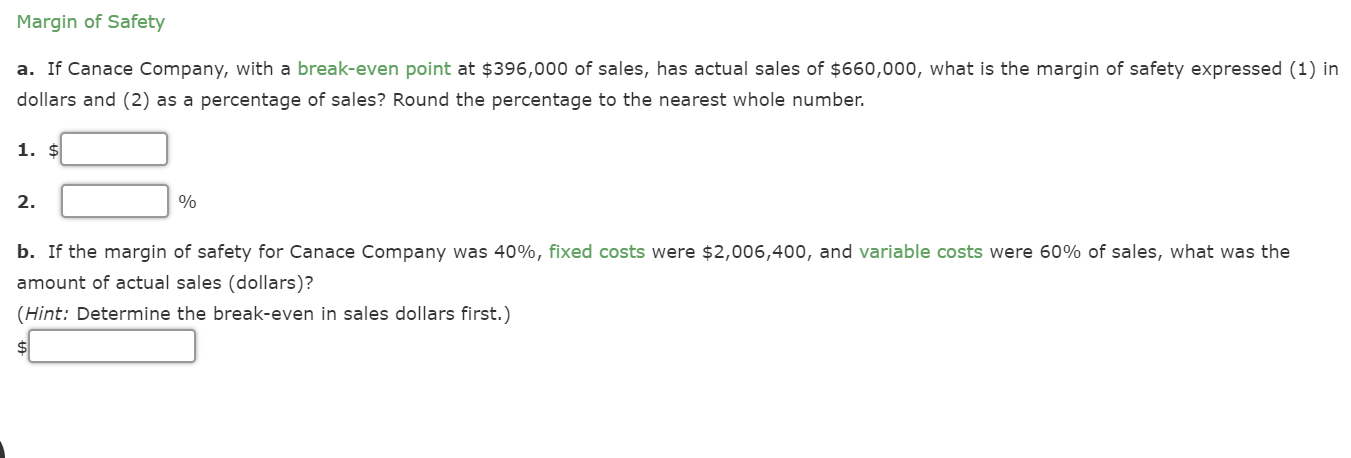Margin of Safety a. If Canace Company, with a break-even point at \$396,000 of sales, has actual sales of \$660,000, what is the margin of safety expressed (1) in dollars and (2) as a percentage of sales? Round the percentage to the nearest whole number. 1. \$ 2. % b. If the margin of safety for Canace Company was 40%, fixed costs were \$2,006,400, and variable costs were 60% of sales, what was the amount of actual sales (dollars)? (Hint:...

• ### Margin of Safety a. If Canace Company, with a break-even point at \$341,600 of sales, has...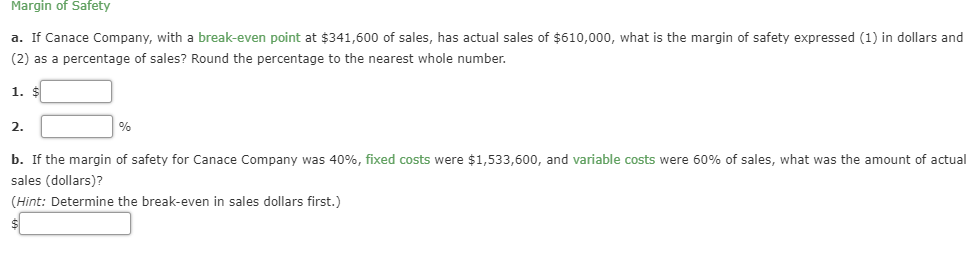Margin of Safety a. If Canace Company, with a break-even point at \$341,600 of sales, has actual sales of \$610,000, what is the margin of safety expressed (1) in dollars and (2) as a percentage of sales? Round the percentage to the nearest whole number 1. \$ 2.1 % b. If the margin of safety for Canace Company was 40%, fixed costs were \$1,533,600, and variable costs were 60% of sales, what was the amount of actual sales (dollars)? (Hint:...

• ### Margin of Safety a. If Canace Company, with a break-even point at \$960,000 of sales, has...

Margin of Safety a. If Canace Company, with a break-even point at \$960,000 of sales, has actual sales of \$1,200,000, what is the margin of safety expressed (1) in dollars and (2) as a percentage of sales? Round the percentage to the nearest whole number. 1. \$ 2.   % b. If the margin of safety for Canace Company was 20%, fixed costs were \$1,875,000, and variable costs were 80% of sales, what was the amount of actual sales (dollars)? (Hint:...

• ### Margin of Safety a. If Canace Company, with a break-even point at \$416,100 of sales, has...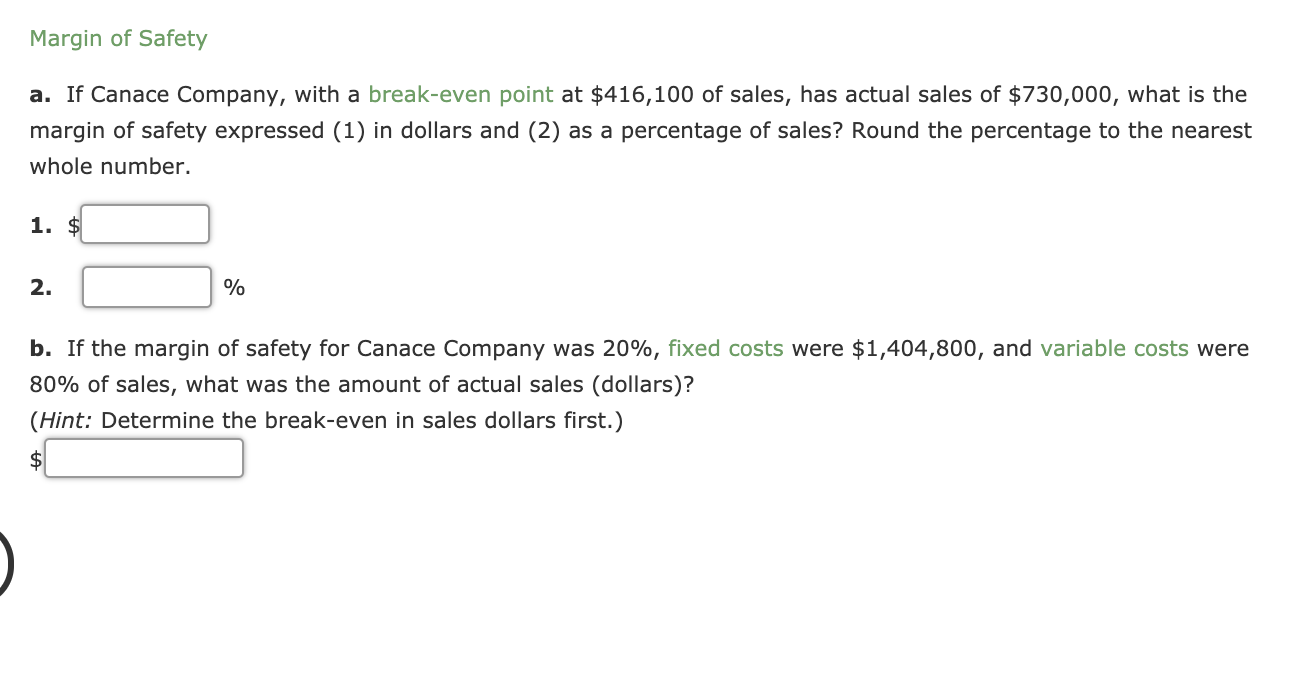Margin of Safety a. If Canace Company, with a break-even point at \$416,100 of sales, has actual sales of \$ 730,000, what is the margin of safety expressed (1) in dollars and (2) as a percentage of sales? Round the percentage to the nearest whole number. 1. \$ 2. % b. If the margin of safety for Canace Company was 20%, fixed costs were \$1,404,800, and variable costs were 80% of sales, what was the amount of actual sales (dollars)?...

• ### Margin of Safety a. If Canace Company, with a break-even point at \$518,000 of sales, has...

Margin of Safety a. If Canace Company, with a break-even point at \$518,000 of sales, has actual sales of \$740,000, what is the margin of safety expressed (1) in dollars and (2) as a percentage of sales? Round the percentage to the nearest whole number. 1. \$ 2.   % b. If the margin of safety for Canace Company was 30%, fixed costs were \$1,633,800, and variable costs were 70% of sales, what was the amount of actual sales (dollars)? (Hint:...

• ### Margin of Safety a. If Canace Company, with a break-even point at \$539,500 of sales, has...

Margin of Safety a. If Canace Company, with a break-even point at \$539,500 of sales, has actual sales of \$650,000, what is the margin of safety expressed (1) in dollars and (2) as a percentage of sales? Round the percentage to the nearest whole number. 1. \$? 2. ? % b. If the margin of safety for Canace Company was 35%, fixed costs were \$1,688,050, and variable costs were 65% of sales, what was the amount of actual sales (dollars)?...

• ### Margin of Safety a. If Canace Company, with a break-even point at \$390,400 of sales, has...

Margin of Safety a. If Canace Company, with a break-even point at \$390,400 of sales, has actual sales of \$640,000, what is the margin of safety expressed (1) in dollars and (2) as a percentage of sales? Round the percentage to the nearest whole number. 1. \$ 2.   % b. If the margin of safety for Canace Company was 20%, fixed costs were \$1,249,600, and variable costs were 80% of sales, what was the amount of actual sales (dollars)? (Hint:...

• ### Margin of Safety a. If Canace Company, with a break-even point at \$448,000 of sales, has...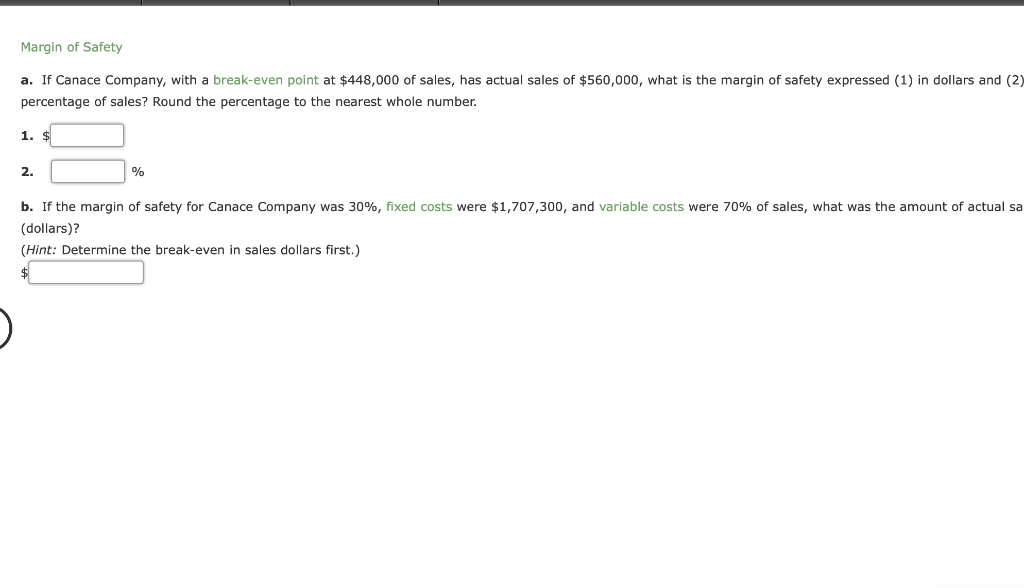Margin of Safety a. If Canace Company, with a break-even point at \$448,000 of sales, has actual sales of \$560,000, what is the margin of safety expressed (1) in dollars and (2) percentage of sales? Round the percentage to the nearest whole number. 1. \$ 2. b. If the margin of safety for Canace Company was 30%, fixed costs were \$1,707,300, and variable costs were 70% of sales, what was the amount of actual sa (dollars)? (Hint: Determine the break-even...

• ### Margin of Safety a. If Canace Company, with a break-even point at \$477,400 of sales, has...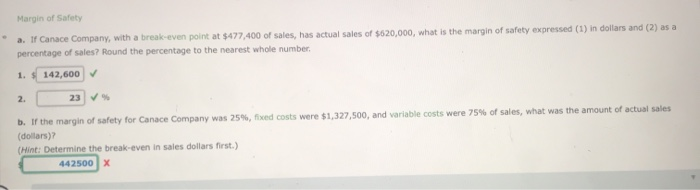Margin of Safety a. If Canace Company, with a break-even point at \$477,400 of sales, has actual sales of \$620,000, what is the margin of safety expressed (1) in dollars and (2) as a percentage of sales? Round the percentage to the nearest whole number, 1. 142,600 2. 23 % b. If the margin of safety for Canace Company was 25%, fixed costs were \$1,327,500, and variable costs were 75% of sales, what was the amount of actual sales (dollars)?...

• ### 9 Margin of Safety a. If Canace Company, with a break-even point at \$312,800 of sales,...

9 Margin of Safety a. If Canace Company, with a break-even point at \$312,800 of sales, has actual sales of \$460,000, what is the margin of safety expressed (1) in dollars and (2) as a percentage of sales? Round the percentage to the nearest whole number. 1. \$ 2.   % b. If the margin of safety for Canace Company was 45%, fixed costs were \$1,655,775, and variable costs were 55% of sales, what was the amount of actual sales (dollars)?...